• 对数几率回归的一个简单的算法描述，可以参看周志华机器学习一书。
• 文章目录一、对数几率和对数几率回归二、Sigmoid函数三、极大似然法四、梯度下降法四、Python实现 一、对数几率和对数几率回归   在对数几率回归中，我们将样本的模型输出y∗y^*y∗定义为样本为正例的概率，将y∗1...
• python代码，实现了OVO对数几率回归, iris.data数据集用OVO方法进行分类，
• 对数几率回归（Logistic Regression），又称为逻辑回归的python实现，并且通过梯度下降法进行优化
• 对数几率回归练习数据，对应的两个txt文件，有测试数据和训练数据，对应的每一行是数据集， 训练集有三列，最后一列是label ，其他两个是特征， 测试集有2列，都是特征
• 文章目录一、对数几率和对数几率回归二、Sigmoid函数三、极大似然法四、梯度下降法四、Python实现 一、对数几率和对数几率回归   在对数几率回归中，我们将样本的模型输出y∗y^*y∗定义为样本为正例的概率，将y∗1...
文章目录一、对数几率和对数几率回归二、Sigmoid函数三、极大似然法四、梯度下降法四、Python实现
一、对数几率和对数几率回归
在对数几率回归中，我们将样本的模型输出$y^*$定义为样本为正例的概率，将$\frac{y^*}{1-y^*}$定义为几率（odds），几率表示的是样本作为正例的相对可能性。将几率取对便可以得到对数几率（log odds，logit）。
$logit=\log\frac{y^*}{1-y^*}$
而对数几率回归（Logistic Regression）则试图从样本集中学得模型$w^Tx$并使其逼近该样本的对数几率，从而可以得到：
$condition1:w^Tx=\log\frac{y^*}{1-y^*}$
二、Sigmoid函数
通过求解$conditoin1$可以得到：
$y^*=\frac{e^{w^Tx}}{1+e^{w^Tx}}=\frac{1}{1+e^{-w^Tx}}$
由此我们可以知道样本$x_i$为正例的概率可以通过函数$h(w^Tx_i)=\frac{1}{1+e^{-w^Tx_i}}$来表示。而其中的函数$h(z)$便被称为Sigmoid函数，其图像如下：求其导数：
$h'(z)=\frac{-e^{-z}}{(1+e^{-z})^2}=\frac{1}{1+e^{-z}}(1-\frac{1}{1+e^{-z}})=h(z)(1-h(z))$
这是一个很好的性质，有利于简化后面优化模型时的计算。
三、极大似然法
通过前面的推导，可以得到：
$P(y=1|x)=y^*=h(w^Tx)\,\,\,\,\,\,\,\,P(y=0|x)=1-y^*=1-h(w^Tx)$
合并两个式子，则有：
$P(y|x)=h(w^Tx)^y(1-h(w^Tx))^{1-y}$
求出了样本标记的分布律，便可以通过极大似然法来估计分布律中的参数$w$。先写出极大似然函数：
$L(y_i|x_i,w)=\prod^{m}_{i=1}h(w^Tx_i)^{y_i}(1-h(w^Tx_i))^{1-{y_i}}$
对极大似然函数取对可以得到对数似然函数：
$l(y_i|x_i,w)=log(L)=\sum^{m}_{i=1}{(y_i\log h(w^Tx_i)+(1-y_i)log(1-h(w^Tx_i)))}$
在前面乘上负数因子便可以得到对数几率回归的代价函数：
$J(w)=-\frac{1}{m}\sum^{m}_{i=1}{(y_i\log h(w^Tx_i)+(1-y_i)log(1-h(w^Tx_i)))}$
通过最小化上述代价函数便可以估计出参数$w$的值。
四、梯度下降法
通过上述步骤，优化对数几率回归模型的关键变成了求解：
$w=\argmin J(w)=\argmin -\frac{1}{m}\sum^{m}_{i=1}{(y_i\log h(w^Tx_i)+(1-y_i)log(1-h(w^Tx_i)))}$
在《线性回归：梯度下降法优化》中，我已经详细介绍了梯度下降法的数学原理，这里直接使用梯度下降法来对对数几率回归模型进行优化。
对$J(w)$进行求导：
$\frac{\partial J}{\partial w}=-\frac{1}{m}\sum^{m}_{i=1}(y_i(1-h(w^Tx_i))x_i+(y_i-1)h(w^Tx_i)x_i)=-\frac{1}{m}\sum^{m}_{i=1}{(y_i-h(w^Tx_i))x_i}$
将$\frac{\partial J}{\partial w}$带入参数$w$的更新公式$w^*=w-\eta\frac{\partial J}{\partial w}$，最终得到$w$的更新公式如下：
$w^*=w+\frac{\eta}{m}\sum^{m}_{i=1}{(y_i-h(w^Tx_i))x_i}$
四、Python实现
梯度下降优化算法：
    def fit(self, X, y):
self.W = np.zeros(X.shape + 1)
for i in range(self.max_iter):
delta = self._activation(self._linear_func(X)) - y
self.W -= self.eta * delta.sum()
self.W[1:] -= self.eta * (delta @ X)
return self

导入鸢尾花数据集进行测试：
if __name__ == "__main__":
from sklearn import datasets
from sklearn.model_selection import train_test_split
from sklearn.metrics import classification_report

irirs = datasets.load_iris()
X = irirs["data"][:100]
y = irirs["target"][:100]
X_train, X_test, y_train, y_test = train_test_split(X, y, train_size=0.7, test_size=0.3)
classifier = LRClassifier().fit(X_train, y_train)
y_pred = classifier.predict(X_test)
print(classification_report(y_test, y_pred))

分类报告如下：展开全文机器学习 python
• 本文内容：对数几率回归 个人总结：本节课主要介绍了对数几率回归，并延伸对比了最大熵与均方误差的区别、判别模型和生成模型的区别、对数几率回归的局限性以及引入深度学习 参考笔记： ... 文章目录1 那么按照机器...
本文内容：对数几率回归
个人总结：本节课主要介绍了对数几率回归，并延伸对比了最大熵与均方误差的区别、判别模型和生成模型的区别、对数几率回归的局限性以及引入深度学习

参考笔记：
https://sakura-gh.github.io/ML-notes/ML-notes-html/2_Regression-Case-Study.html
https://piperliu.blog.csdn.net/article/details/107456428

文章目录1 那么按照机器学习三步骤：Step1: function setStep 2：Goodness of a functionstep 3：Find the best function2 Logistic Regression V.s. Linear Regression3 Logistic Regression + Square error？4 Discriminative v.s. Generative5 Multi-class Classificationsoftmax 处理过程那么为什么是e呢，不是别的函数？6 Limitation of Logistic Regression解决办法： Feature Transformation实例举例7 Powerful Cascading Logistic Regression
上一节中我们最后利用概率模型来计算最后的分类结果，对于新样本点可通过
$P\left(C_{1} \mid x\right)=\frac{P\left(C_{1}\right) P\left(x \mid C_{1}\right)}{P\left(C_{1}\right) P\left(x \mid C_{1}\right)+P\left(C_{2}\right) P\left(x \mid C_{2}\right)}$
来预测结果是class1 还是 class2，并且我们通过logistic function，将w*x值由实数值转化为逻辑值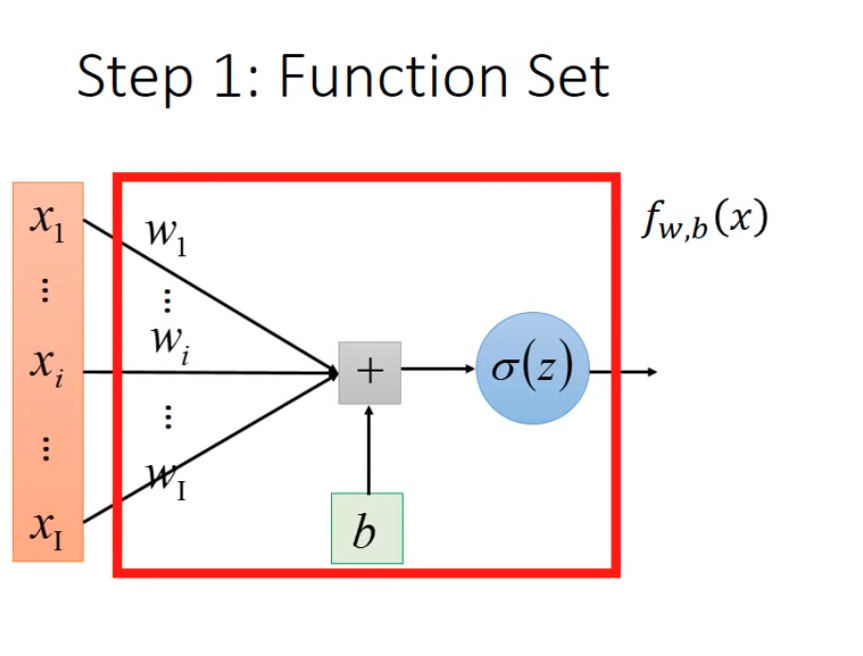1 那么按照机器学习三步骤：
Step1: function set
这里的函数集即为对数几率回归函数集
$z=\sum_{i} w_{i} x_{i}+b$
Step 2：Goodness of a function
$w^{*}, b^{*}=\arg \max _{w, b} L(w, b)=\arg \min _{w, b}\left(-\ln L(w, b)=\sum_{n}-\left[\hat{y}^{n} \ln f_{w, b}\left(x^{n}\right)+\left(1-\hat{y}^{n}\right) \ln \left(1-f_{w, b}\left(x^{n}\right)\right)\right]\right.$
step 3：Find the best function
发现最适合的function也就是对以上这个目标式求最优解
2 Logistic Regression V.s. Linear Regression
3 Logistic Regression + Square error？
根据Square Error目标式的梯度计算可以发现：
$f_{w, b}(x)-\hat{y}$
当
$f_{w, b}\left(x^{n}\right)=0$
这一项等于0时，梯度为0，但是却离target很远，并没有达到预期要求。所以可能在梯度求导过程中出现卡顿、缓慢等现象。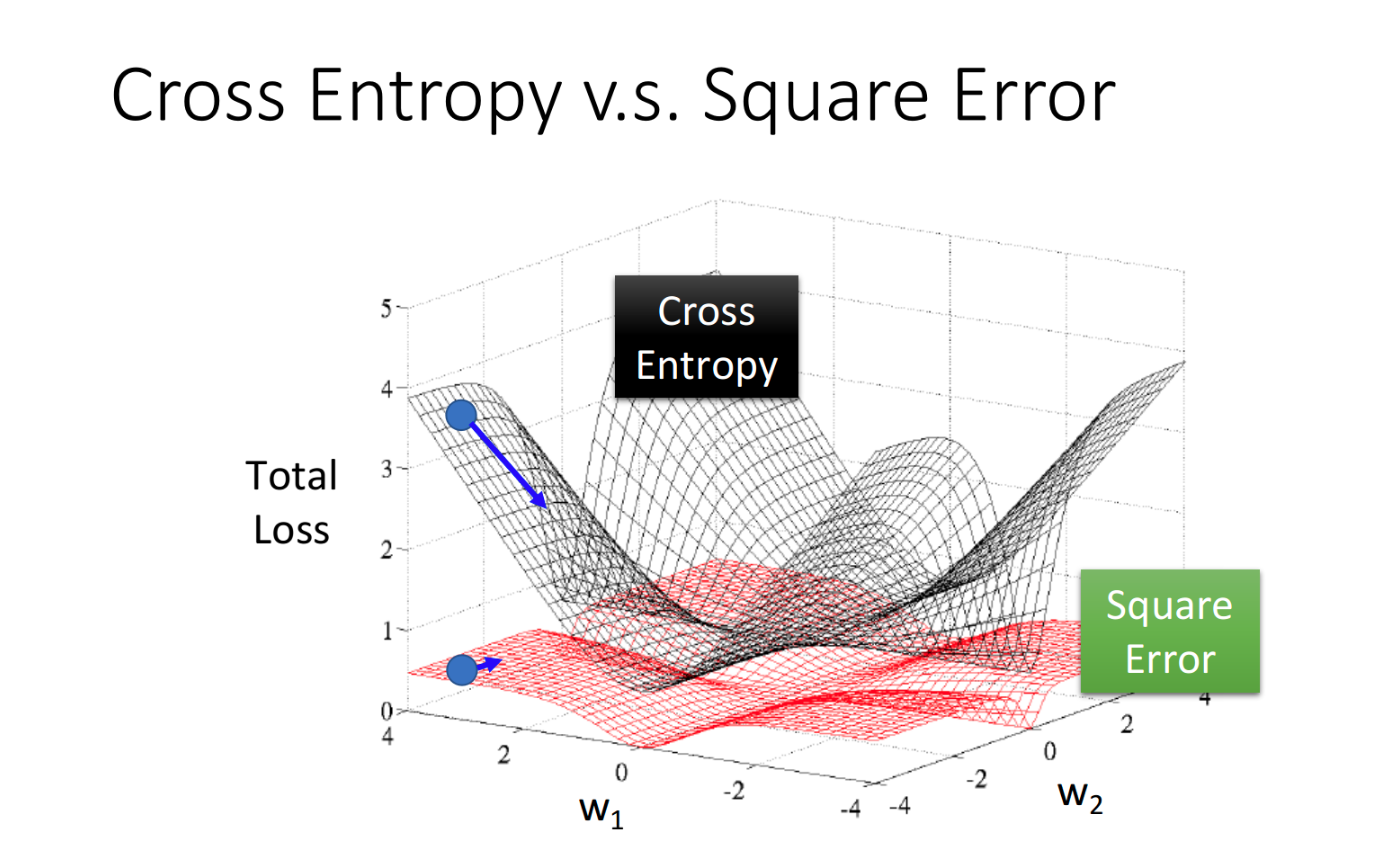4 Discriminative v.s. Generative
而在Generative model里面，我们对Probability distribution是有实质性的假设的，之前我们假设的是Gaussian(高斯分布)，甚至假设在相互独立的前提下是否可以是naive bayes(朴素贝叶斯)，也就是Generative model中定义好了一个space，我们所求的w,b只是其中的一小部分。
但是Discriminative则是只根据当前的data 信息进行判断，没有作相应的假设。
5 Multi-class Classification
softmax 处理过程
对大的值做强化：这件事情呢叫softmax
那么为什么是e呢，不是别的函数？
因为也可以换成别的，。。。yi…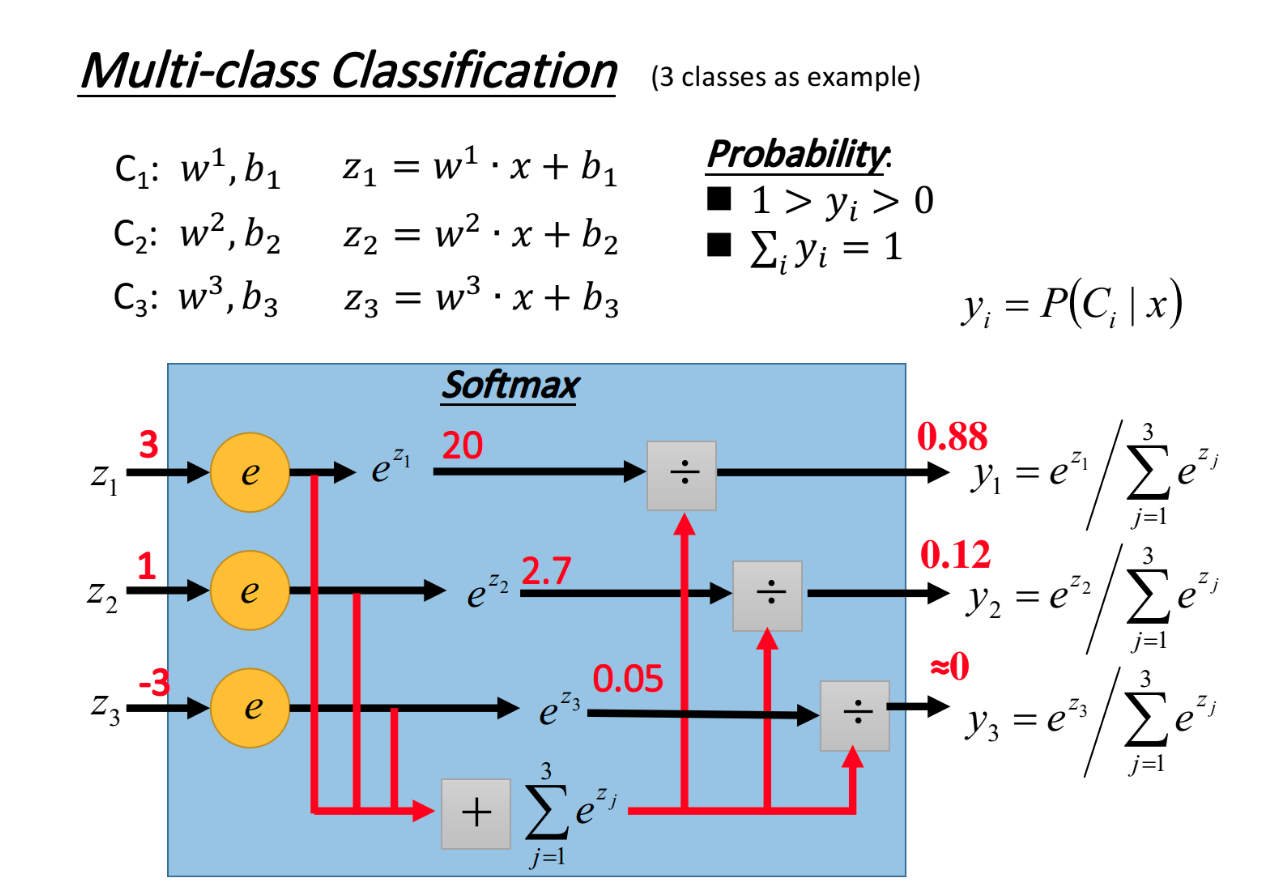大家也可以通过最大熵模型进行切入，最后得到的也是和softmax一致的结果
极大似然估计法得到最终的交叉熵表达式的
6 Limitation of Logistic Regression
对数几率回归无法解决异或问题：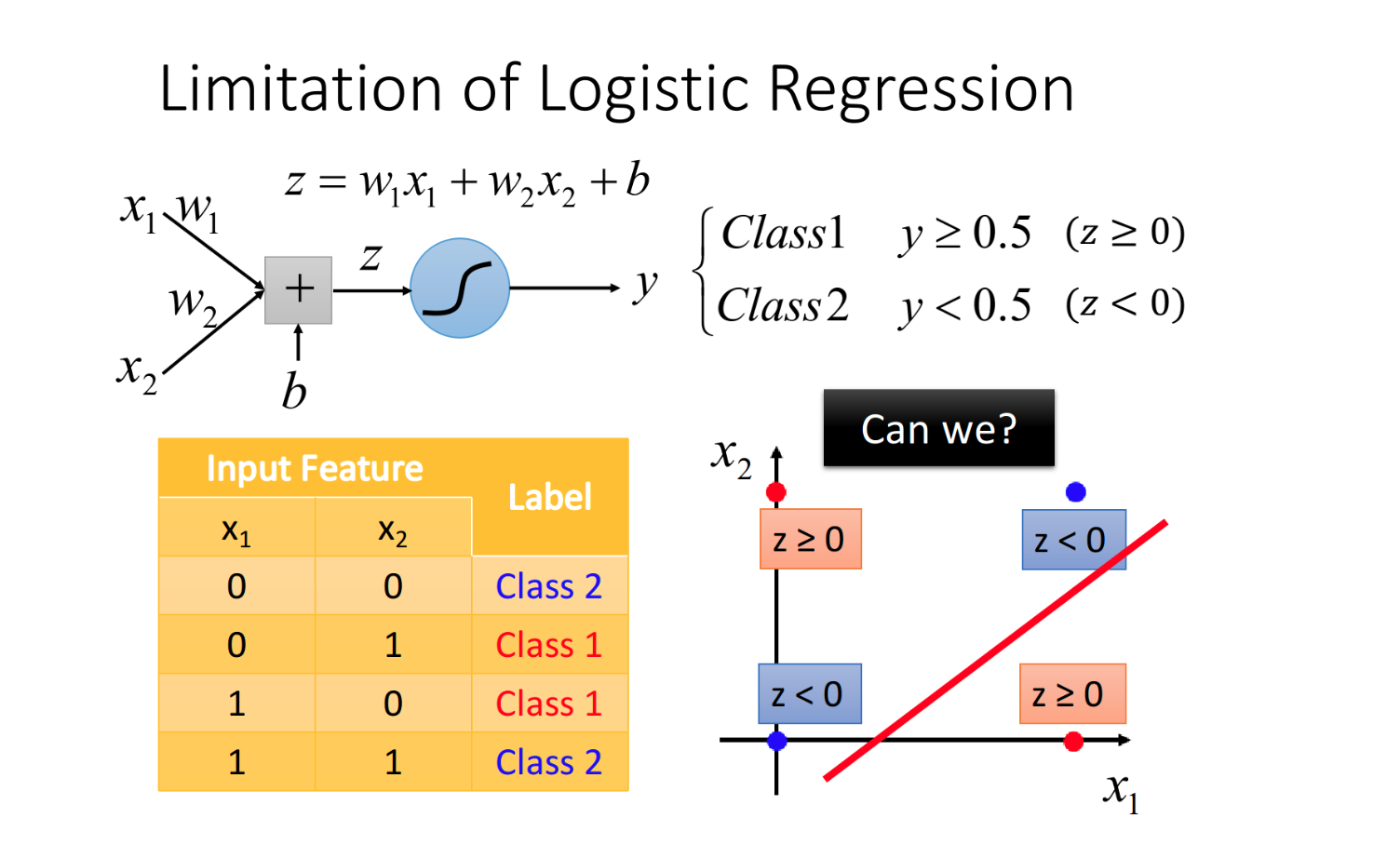解决办法： Feature Transformation
这里计算原来点到[0,0]和[1,1]之间的距离，是计算新向量内，每个元素对应到这两个点的距离
[0,0]  [0 sqrt(2)]
[0,1]  [1,1]
[1,0]  [1,1]
[1,1]  [sqrt(2),0]
模型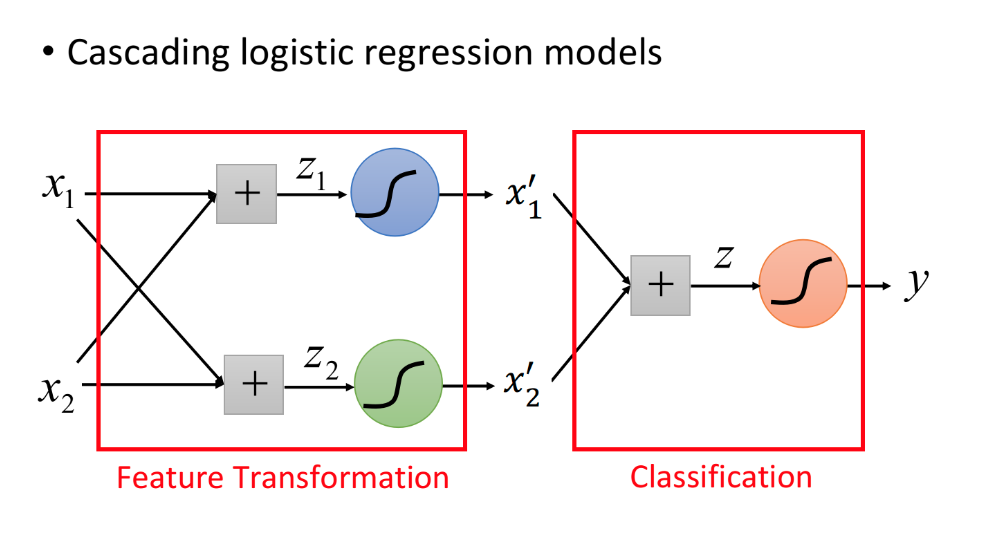让机器自己找特征转换
我们可以让很多Logistic Regression cascade(连接)起来
实例举例
将原来的异或问题，转化为可分的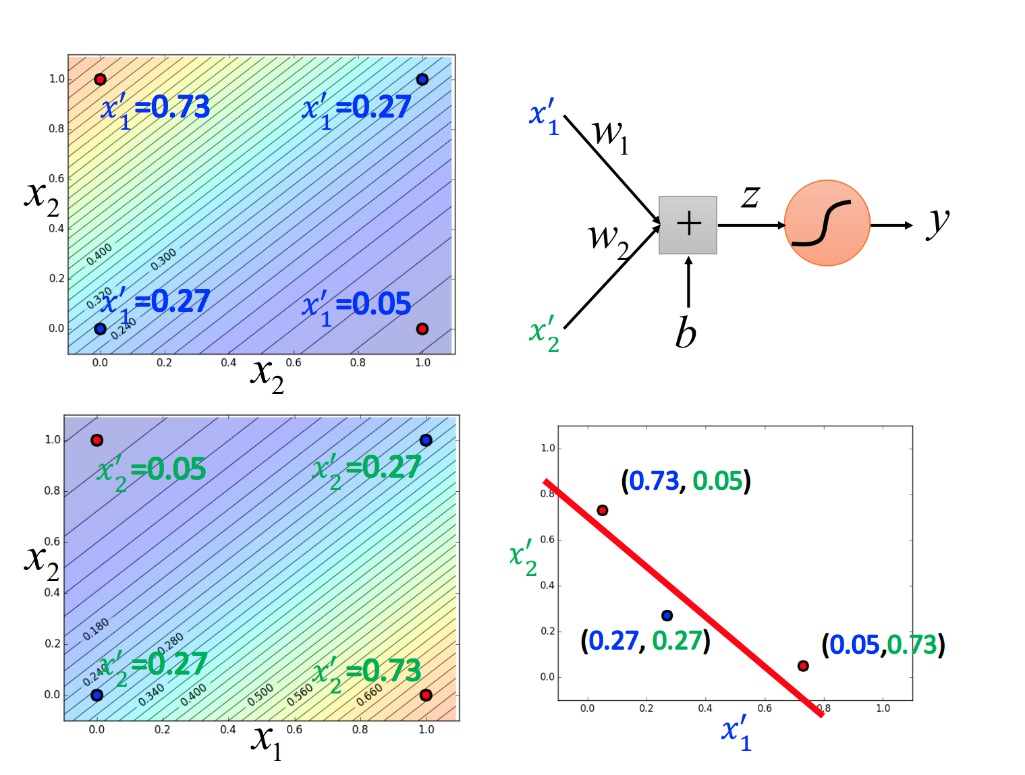7 Powerful Cascading Logistic Regression
当把多个这样的对数几率回归串起来的网络有了一个新的名字：深度神经网络
所以后来就有了很多故事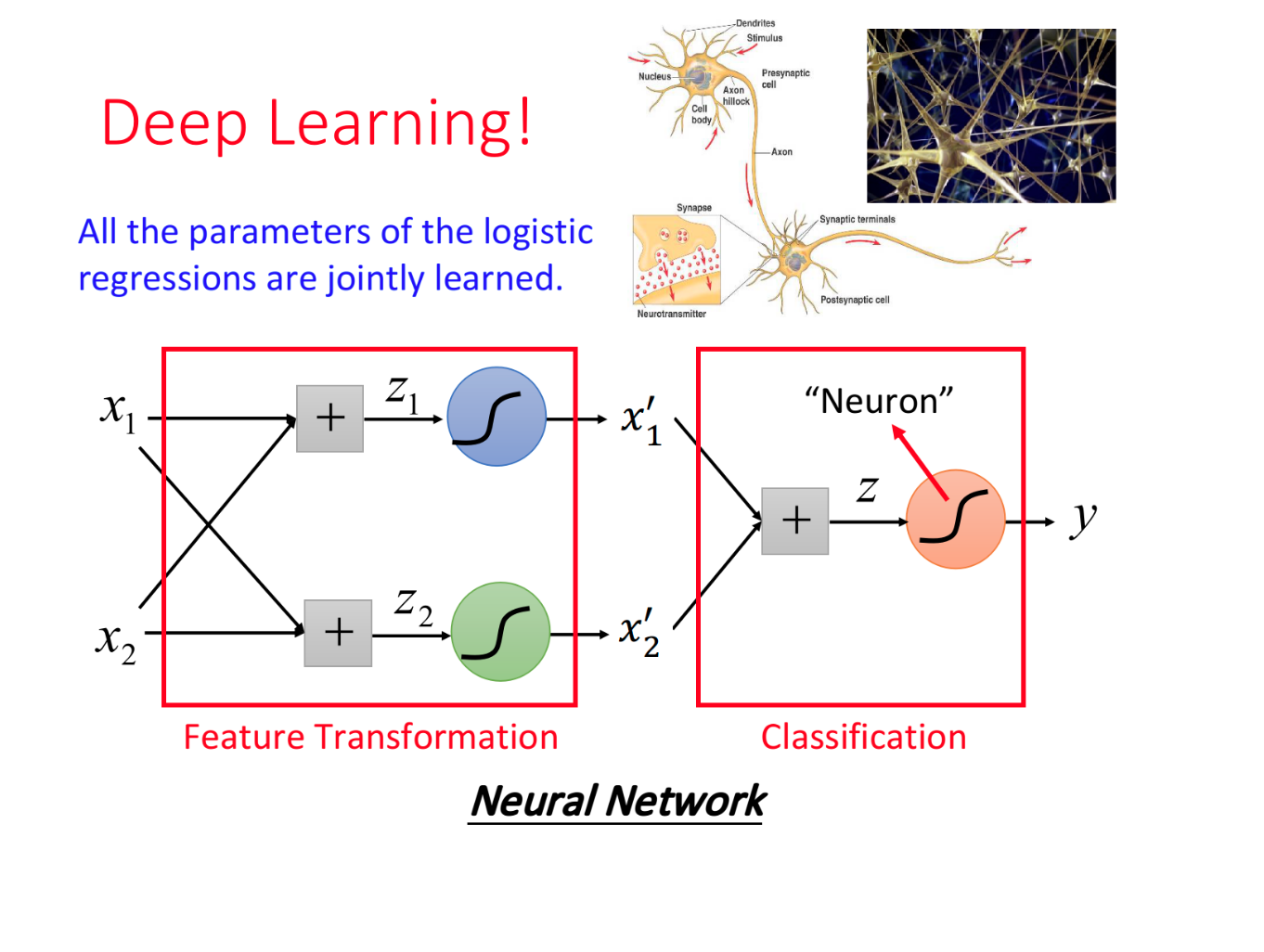展开全文• 什么是对数几率回归 logistic regression:中文有多种译法，例如：逻辑回归（比较常见），对数几率回归（周志华）。 对数几率回归是一种常用的分类算法，例如二分类，多分类等。 如何进行对数几率回归 对于二分类...
什么是对数几率回归
	logistic regression:中文有多种译法，例如：逻辑回归（比较常见），对数几率回归（周志华）。
对数几率回归是一种常用的分类算法，例如二分类，多分类等。

如何进行对数几率回归
	对于二分类，结果只有y=0|1两种，可以使用阶跃函数来描述其输出：
h(x)=1 p>0.5
h(x)=0 p<0.5
由于这个函数不连续，所以通常使用某个连续函数使得h(x)的结果位于0-1之间，而我们通常选用的就
是sigmoid函数，也叫logistic函数。上图就是sigmoid函数的曲线，对于线性回归函数进行sigmoid变换即可得逻辑回归函数，此时离散的问题化为连续的线性回归。
为什么进行对数几率回归
	对数几率回归因为比较简单常用于文本分类，癌症检测等学习问题上，通过sigmoid变换后的结果是连
续的，因而可以得到相应的概率值。



展开全文• 1.对数几率回归 本质是一个神经元。 对数几率回归可以回答Yes-No类的问题。 logistic函数，亦称为sigmod函数。 f(x)=1/(1+exp(-x)) sigmod函数是一个概率分布函数,即给定某个特定输入，该函数将计算输出为“success...
1.对数几率回归
本质是一个神经元。
对数几率回归可以回答Yes-No类的问题。
logistic函数，亦称为sigmod函数。
f(x)=1/(1+exp(-x))
sigmod函数是一个概率分布函数,即给定某个特定输入，该函数将计算输出为“success”的概率。
sigmod函数接收单个输入值。对于多维数据，需要将他们合并为单个值，可利用前面的线性回归模型表达式。

-*-牢记：sigmod函数的输出是一个概率值。
2.预测部份代码
#与对数几率回归相同的参数和变量初始化
W = tf.Variable(tf.zeros([5, 1]),name="weights")
b = tf.Variable(0, name="bias")

#之前的推断现在用于值的合并
def combine_inputs(X):
return tf.matul(X, W) + b

#新的推断值是将sigmod函数运用到前面的合并值的输出
def inference(X):
return tf.sigmod(combine_inputs(X))

3.损失函数
－＊－交叉熵的原理，详情见：https://blog.csdn.net/tsyccnh/article/details/79163834。
交叉熵可表示预测输出跟期望输出的接近程度，越接近，交叉熵越小。
（1）对于单分类问题
单分类问题：这里的单类别是指，每一张图像样本只能有一个类别，比如只能是狗或只能是猫，单类别的标签是one-hot。
loss = -[p1log(q1) + p2log(q2) + … + pn*log(qn)]
其中pi为期望输出概率，qi为预测输出概率，n为1个样本可能的输出数目。
（2）对于多分类问题
多分类问题：这里的多类别是指，每一张图像样本可以有多个类别，比如同时包含一只猫和一只狗 ，和单分类问题的标签不同，多分类的标签是n-hot。


展开全文...